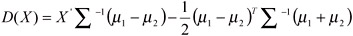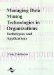# Overview of Discriminant Analysis and Genetic Algorithm Approaches to Discriminant Analysis

 < Day Day Up >

Parametric linear discriminant analysis (LDA) was developed by Fisher (1936). The LDA procedure constructs a linear discriminant function by maximizing the ratio of between-groups variance to within-groups variances. For a binary classification problem, the discriminant function can be written as follows:where μ1, μ2, and -1 are mean vectors for group 1, group 2 and inverse of common covariance matrix respectively. LDA works well when normality and equal dispersion of two groups assumptions are met. However, in reality these assumptions are violated frequently and non-parametric approaches such as GA and neural networks are reported to perform better.

Heuristic genetic algorithms provide a popular nonparametric approach for classification when minimizing misclassification is considered as a performance metric. Genetic algorithms (GAs) use survival of the fittest strategy to learn coefficients of a linear discriminant function. GAs are parallel search techniques that start with a set of random potential solutions and use special search operators (evaluation, selection, crossover, mutation) to bias the search towards the promising solutions. At any given time, unlike any optimization approach, GA has several promising potential solutions (equal to population size) as opposed to one optimal solution. Each population member in a GA is a potential solution. A population member (P1) used to learn the coefficients for a linear discriminant function will consist of a set of all the coefficients and the intercept. P1 can be represented as,

• P1 = w1, w2, w3, w4, c

• Where, (wl, w2, w3, w4, c)

The discriminant function takes the following form,

• w1x1 +w2x2 +w3x3 +w4x4 + c = 0

The classification heuristic can be represented as,

• IF w1x1 + w2x2 + w3x3 + w4x4 + c 0 Then class=G1

• ELSE class = G2

Any w p1 is called a gene (coefficient) of a given population member P1. A set of several population members is called the population Ω. The cardinality of the set of population members Ω (number of population members) is called population size. The cardinality of a population member (number of genes) is called the defining length of the population member ζ. The defining length for population member P1, ζ=5. The defining length of all the population members in a given population is constant.

GA starts with a random set of the population. An evaluation operator is then applied to evaluate the fitness of each individual. In case of learning coefficients for a discriminantfunction, the evaluation function is the number of correctly classified cases. A selection operator is then applied to select the population members with higher fitness (so that they can be assigned a higher probability of survival). Under the selection operator, individual population members may be born, allowed to live, or to die. Several selection operators are reported in the literature; they are proportionate reproduction, ranking selection, tournament selection, and steady state selection (Goldberg & Deb, 1991). Among the popular selection operators are ranking and tournament selection. Goldberg and Deb (1991) show that both ranking and tournament selection maintain strong population fitness growth potential under normal conditions. However, the tournament selection operator requires lower computational overhead. The time complexity of ranking selection is O (nlog n), whereas the time complexity of tournament selection is O(n), where n is the number of members in a population. In tournament selection two random individuals are selected and the member with the better fitness of the two is admitted to the pool of individuals for further genetic processing. The process is repeated in a way such that the population size remains constant and the best individual in the population always survives. We use a tournament selection operator in this research.

After the selection operator is applied, the new population special operator called crossover and mutation is applied with a certain probability. For applying the crossover operator, the status of each population member is determined and each is assigned a status as a survivor or a non-survivor. The number of population members in survivor status is approximately equal to population size* (1-probability of crossover). The number of non-surviving members is approximately equal to population size*probability of crossover. The non-surviving members in a population are then replaced by applying crossover operators to randomly selected surviving members. Though several crossover operators exist we describe and use a one-point crossover operator in our research.

In one-point crossover, two surviving parents and a crossover point are randomly selected. For each parent, the genes in the right-hand side of the crossover point are exchanged to produce two children. Let P1 and P2 be two parents and the crossover point be denoted by "|". The two children C1 and C2 are produced as follows (we use the bold font to simplify the understanding),

• P1 = w1, w2, | w3, w4, c

• P2 = w1, w2, | w3, w4, c

• C1 = w1, w2, | w3, w4, c

• C2 = w1, w2, | w3, w4, c

The mutation operator randomly picks a gene in a surviving population member (with the probability equal to probability of mutation) and replaces it with a real random number.

 < Day Day Up >Managing Data Mining Technologies in Organizations: Techniques and Applications
ISBN: 1591400570
EAN: 2147483647
Year: 2003
Pages: 174